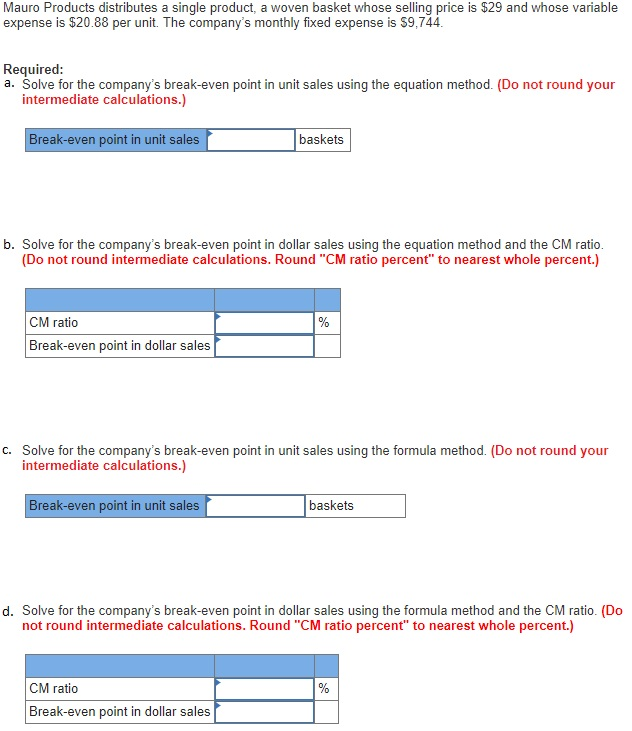# Question & Answer: Mauro Products distributes a single product, a woven basket whose selling price is \$29 and whose variable expense is \$20.88 per unit……Mauro Products distributes a single product, a woven basket whose selling price is \$29 and whose variable expense is \$20.88 per unit. The company’s monthly fixed expense is \$9, 744. Required: a. Solve for the company’s break-even point in unit sales using the equation method. (Do not round your intermediate calculations.) b. Solve for the company’s break-even point in dollar sales using the equation method and the CM ratio. (Do not round intermediate calculations. Round “CM ratio percent” to nearest whole percent.) c. Solve for the company’s break-even point in unit sales using the formula method. (Do not round your intermediate calculations.) d. Solve for the company’s break-even point in dollar sales using the formula method and the CM ratio. (Do not round intermediate calculations. Round “CM ratio percent” to nearest whole percent.)

Don't use plagiarized sources. Get Your Custom Essay on
Question & Answer: Mauro Products distributes a single product, a woven basket whose selling price is \$29 and whose variable expense is \$20.88 per unit……
GET AN ESSAY WRITTEN FOR YOU FROM AS LOW AS \$13/PAGE

Profit = (Units Contribution Margin * Quantity) – Fixed Expenses
Units Contribution Margin = Unit Sale Price – Unit Variable Cost
Units Contribution Margin = \$29 – \$20.88
Units Contribution Margin = \$8.12

At Break Even Point, the Profit will be Zero. Therefore,
\$0 = (\$8.12 * Quantity) – \$9,744
\$9,744 = \$8.12 * Quantity
Quantity = 1,200 units

Contribution Margin Ratio (CM ratio) = Contribution Margin / Sales * 100
Contribution Margin Ratio (CM ratio) = 8.12 / 29 * 100
Contribution Margin Ratio (CM ratio) = 28%

As per Equation Method,
Profit = (CM ratio * Sales) – Fixed Expenses
\$0 = (0.28 * Sales) – \$9,744
\$9,744 = 0.28 * Sales
Sales = \$34,800

As per Formulae method,
Break Even Point (in units) = Fixed Cost / Contribution Margin per unit
Units Contribution Margin = Unit Sale Price – Unit Variable Cost
Units Contribution Margin = \$29 – \$20.88
Units Contribution Margin = \$8.12

Break Even Point (in units) = 9,744 / 8.12
Break Even Point (in units) = 1,200 units